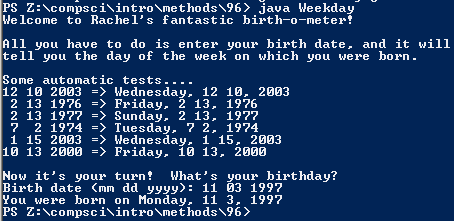# Assignemnt #96: Weekday Calculator

## Code

```    /// Name: Cassie
/// Period: 6
/// Program Name: Weekday Calculator
/// File Name: Weekday.java
/// Date Finished: 3/4/2016

import java.util.Scanner;

public class Weekday
{
public static void main( String[] args )
{
Scanner keyboard = new Scanner(System.in);

System.out.println("Welcome to Rachel's fantastic birth-o-meter!");
System.out.println();
System.out.println("All you have to do is enter your birth date, and it will");
System.out.println("tell you the day of the week on which you were born.");
System.out.println();
System.out.println("Some automatic tests....");
System.out.println("12 10 2003 => " + weekdays(12,10,2003));
System.out.println(" 2 13 1976 => " + weekdays(2,13,1976));
System.out.println(" 2 13 1977 => " + weekdays(2,13,1977));
System.out.println(" 7  2 1974 => " + weekdays(7,2,1974));
System.out.println(" 1 15 2003 => " + weekdays(1,15,2003));
System.out.println("10 13 2000 => " + weekdays(10,13,2000));
System.out.println();

System.out.print("Birth date (mm dd yyyy): ");
int mm = keyboard.nextInt();
int dd = keyboard.nextInt();
int yyyy = keyboard.nextInt();

System.out.println("You were born on " + weekdays(mm, dd, yyyy) );
}

public static String month_name( int month )
{
String result = "error";

if ( month == 1 ) {
result = "January";
}

else if ( month == 2 ) {
result = "February";
}

else if ( month == 3 ) {
result = "March";
}

else if ( month == 4 ) {
result = "April";
}

else if ( month == 5 ) {
result = "May";
}

else if ( month == 6 ) {
result = "June";
}

else if ( month == 7 ) {
result = "July";
}

else if ( month == 8 ) {
result = "August";
}

else if ( month == 9 ) {
result = "September";
}

else if ( month == 10 ) {
result = "October";
}

else if ( month == 11 ) {
result = "November";
}

else if ( month == 12 ) {
result = "December";
}

return result;
}

public static int monthOffset( int month )
{
int result = 1;

if ( month == 1 || month == 10 ) {
result = 1;
}

else if ( month == 2 || month == 3 || month == 11 ) {
result = 4;
}

else if ( month == 4 || month == 7 ) {
result = 0;
}

else if ( month == 9 || month == 12 ) {
result = 6;
}

else if ( month == 5 ) {
result = 2;
}

else if ( month == 6 ) {
result = 5;
}

else if ( month == 8 ) {
result = 3;
}

return result;
}

public static boolean isLeap( int yyyy )
{
// years which are evenly divisible by 4 are leap years,
// but years divisible by 100 are not leap years,
// though years divisible by 400 are leap years
boolean result;

if ( yyyy%400 == 0 )
result = true;
else if ( yyyy%100 == 0 )
result = false;
else if ( yyyy%4 == 0 )
result = true;
else
result = false;

return result;
}

public static String weekday_name(int yyyy, int mm, int dd)
{
int yy, total, day;
String date = "";
yy = yyyy - 1900;
total = yy/ 4 + yy + monthOffset(mm) + dd;

if ( isLeap(yyyy) == true && mm == 1 )
total = total - 1;

else if ( isLeap(yyyy) == true && mm ==2 )
total = total -1 ;

// bunch of calculations go here

String Day;
day = total % 7;

if ( day == 1 )
Day = "Sunday";
else if ( day == 2 )
Day = "Monday";
else if ( day == 3 )
Day = "Tuesday";
else if ( day == 4 )
Day = "Wednesday";
else if ( day == 5 )
Day = "Thursday";
else if ( day == 6 )
Day = "Friday";
else if ( day == 7 )
Day = "Saturday";
else
Day = "error";

return Day;
}

public static String weekdays( int mm, int dd, int yyyy )
{
int yy, total, day;
String date = "";
yy = yyyy - 1900;
total = yy / 4 + yy + monthOffset(mm) + dd;

if ( isLeap(yyyy) == true && mm == 1 ) {
total = total - 1;
}

else if ( isLeap(yyyy) == true && mm == 2 ) {
total = total - 1;
}

day = total % 7;

date = weekday_name(yyyy,mm,dd) + ", " + mm + " " + dd + ", " + yyyy;

return date;
}
}

```

### Picture of the output